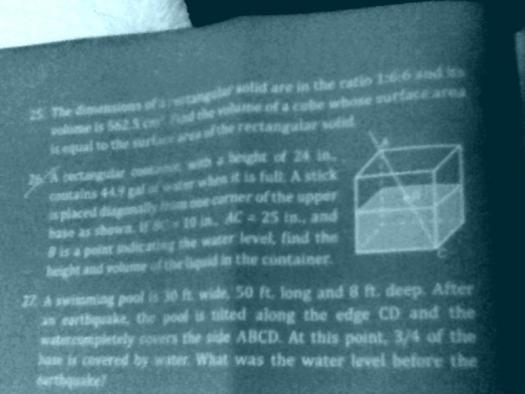# Rectangular

2 posts / 0 new
MichMagandaRectangular

A rectangular container, with a height of 24 in., contains 44.9 gal of water when it is full. A stick is placed diagonally from one corner of the upper base as shown. If BC = 10in., AC= 25in. andis a point indicating the water level, find the height and volume of the liquid in the container.

Rate:
Jhun Vert$\dfrac{h_w}{BC} = \dfrac{24}{AC}$

$\dfrac{h_w}{10} = \dfrac{24}{25}$

$h_w = 9.6 ~ \text{in.}$

When full
$V = A_b \times h$

$44.9 ~ \text{gal} \left( \dfrac{231 ~ \text{in.}^3}{1 ~ \text{gal}} \right) = A_b \times 24$

$A_b = 432.1625 ~ \text{in.}^2$

Volume of water
$V_w = A_b \times h_w$

$V_w = 432.1625 \times 9.6$

$V_w = 4148.76 ~ \text{in.}^3$

$V_w = 4148.76 ~ \text{in.}^3 \left( \dfrac{1 ~ \text{gal}}{231 ~ \text{in.}^3} \right)$

$V_w = 17.96 ~ \text{gal}$

Rate: